Next: Motion in a general Up: Conservation of energy Previous: Potential energy

## Hooke's law

Consider a masswhich slides over a horizontal frictionless surface. Suppose that the mass is attached to a light horizontal spring whose other end is anchored to an immovable object. See Fig. 42. Letbe the extension of the spring: i.e., the difference between the spring's actual length and its unstretched length. Obviously,can also be used as a coordinate to determine the horizontal displacement of the mass. According to Hooke's law, the forcethat the spring exerts on the mass is directly proportional to its extension, and always acts to reduce this extension. Hence, we can write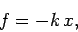(159)

where the positive quantityis called the force constant of the spring. Note that the minus sign in the above equation ensures that the force always acts to reduce the spring's extension: e.g., if the extension is positive then the force acts to the left, so as to shorten the spring.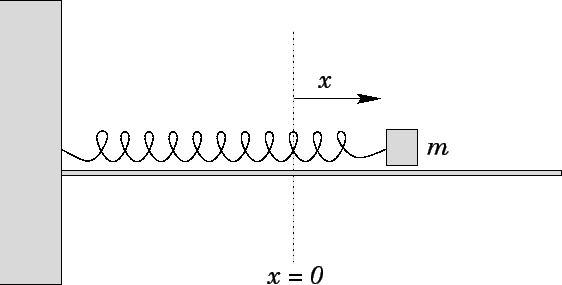According to Eq. (140), the work performed by the spring force on the mass as it moves from displacement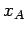to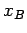is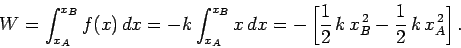(160)

Note that the right-hand side of the above expression consists of the difference between two factors: the first only depends on the final state of the mass, whereas the second only depends on its initial state. This is a sure sign that it is possible to associate a potential energy with the spring force. Equation (155), which is the basic definition of potential energy, yields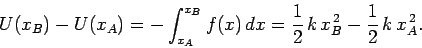(161)

Hence, the potential energy of the mass takes the form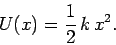(162)

Note that the above potential energy actually represents energy stored by the spring--in the form of mechanical stresses--when it is either stretched or compressed. Incidentally, this energy must be stored without loss, otherwise the concept of potential energy would be meaningless. It follows that the spring force is another example of a conservative force.

It is reasonable to suppose that the form of the spring potential energy is somehow related to the form of the spring force. Let us now explicitly investigate this relationship. If we let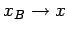and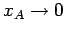then Eq. (161) gives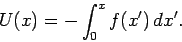(163)

We can differentiate this expression to obtain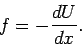(164)

Thus, in 1-dimension, a conservative force is equal to minus the derivative (with respect to displacement) of its associated potential energy. This is a quite general result. For the case of a spring force: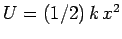, so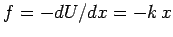.

As is easily demonstrated, the 3-dimensional equivalent to Eq. (164) is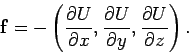(165)

For example, we have seen that the gravitational potential energy of a massmoving above the Earth's surface is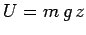, wheremeasures height off the ground. It follows that the associated gravitational force is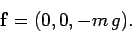(166)

In other words, the force is of magnitude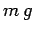, and is directed vertically downward.

The total energy of the mass shown in Fig. 42 is the sum of its kinetic and potential energies: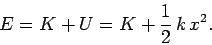(167)

Of course,remains constant during the mass's motion. Hence, the above expression can be rearranged to give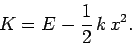(168)

Since it is impossible for a kinetic energy to be negative, the above expression suggests that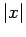can never exceed the value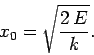(169)

Here,is termed the amplitude of the mass's motion. Note that whenattains its maximum value, or its minimum value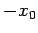, the kinetic energy is momentarily zero (i.e.,).Next: Motion in a general Up: Conservation of energy Previous: Potential energy
Richard Fitzpatrick 2006-02-02